Question

# An I-section has the dimensions shown in Fig. Q4 and is subjected to an axial torque,...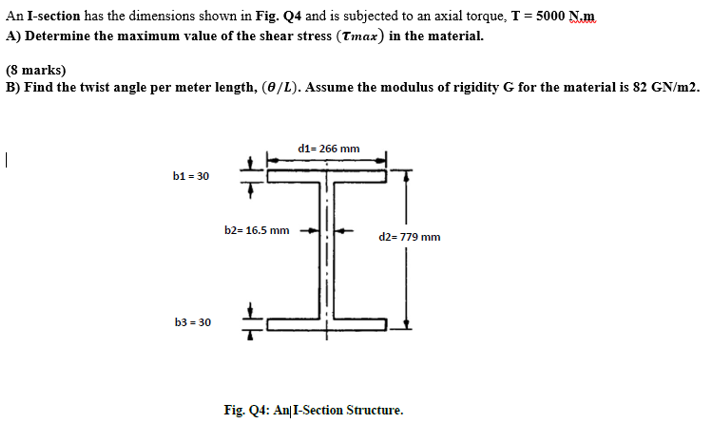An I-section has the dimensions shown in Fig. Q4 and is subjected to an axial torque, T = 5000 Nm A) Determine the maximum value of the shear stress (Tmax) in the material. (8 marks) B) Find the twist angle per meter length, (0/L). Assume the modulus of rigidity G for the material is 82 GN/m2. d1= 266 mm — b1 = 30 b2= 16.5 mm d2= 779 mm b3 = 30 Fig. Q4: An I-Section Structure.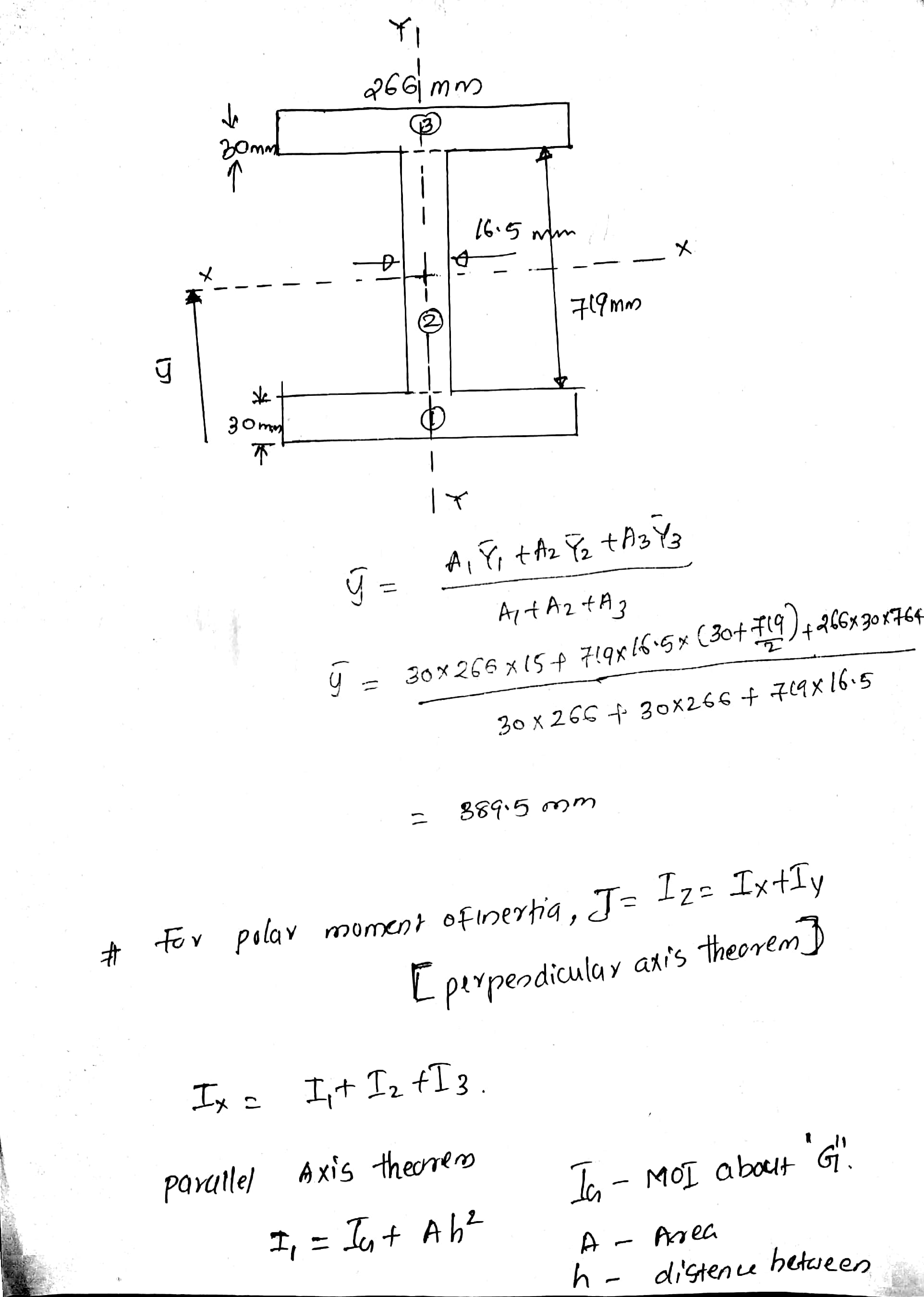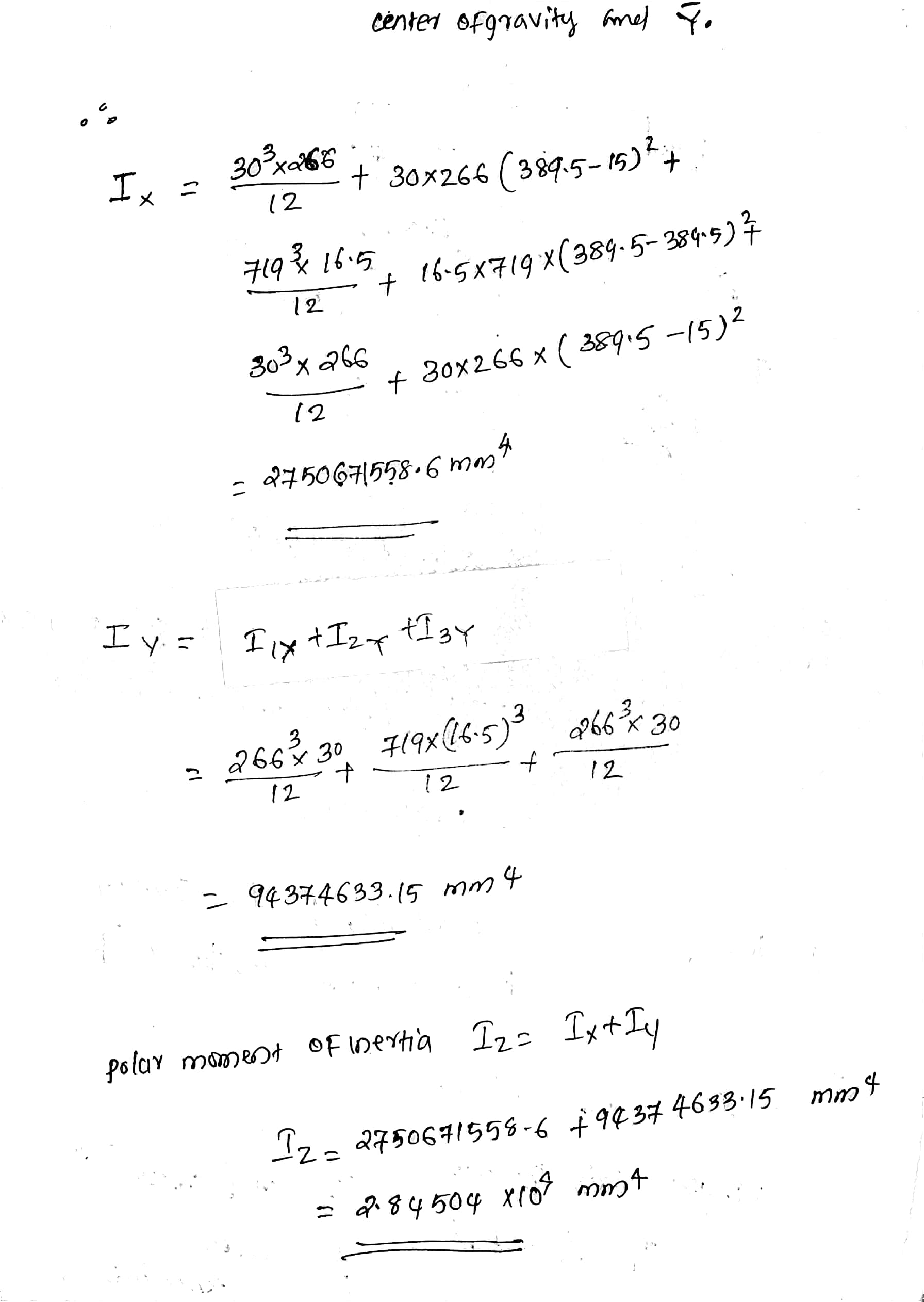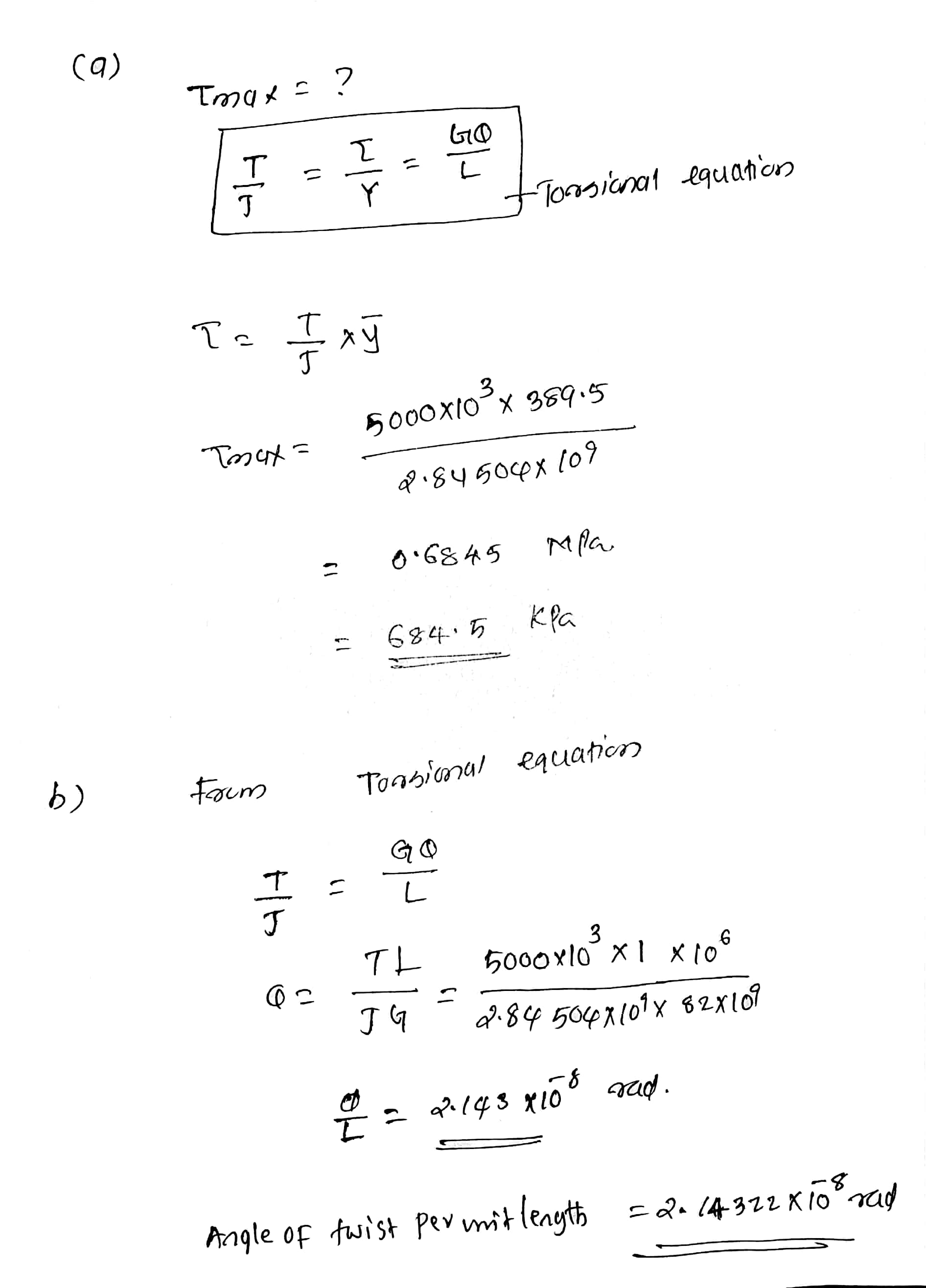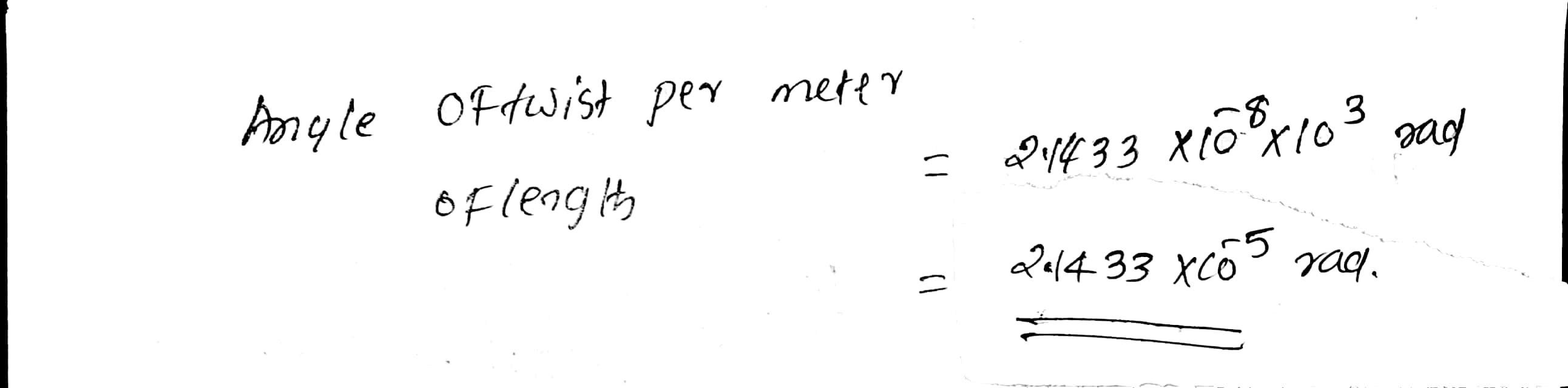#### Earn Coins

Coins can be redeemed for fabulous gifts.

Similar Homework Help Questions
• ### A bar ABCD with varying cross section and subjected to axial loads is shown schematically in...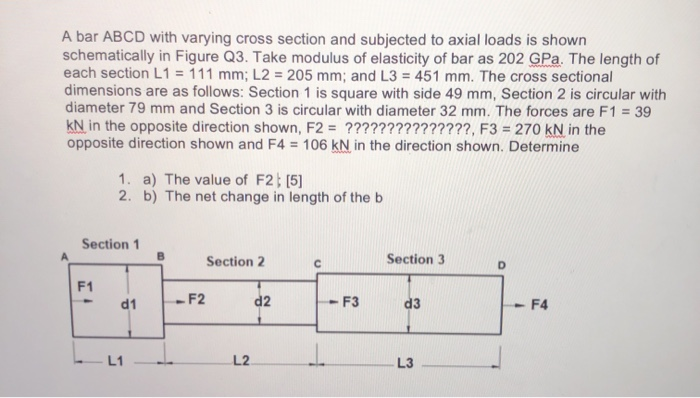A bar ABCD with varying cross section and subjected to axial loads is shown schematically in Figure Q3. Take modulus of elasticity of bar as 202 GPa. The length of each section L1 = 111 mm; L2 = 205 mm; and L3 = 451 mm. The cross sectional dimensions are as follows: Section 1 is square with side 49 mm, Section 2 is circular with diameter 79 mm and Section 3 is circular with diameter 32 mm. The forces are...

• ### 1. A closely coiled helical spring made of 15 mm diameter steel wire has 15 coils...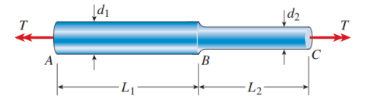1. A closely coiled helical spring made of 15 mm diameter steel wire has 15 coils of 110 mm mean diameter. The spring is subjected to an axial load of 180 N. Calculate the maximum shear stress induced in the spring. Assume modulus of rigidity is 78 GPa. 2. A solid circular bar ABC consists of two segments, as shown in the figure. One segment has diameter d1= 65 mm and length L1 = 1.54 m; the other segment has...

• ### A strip of high-strength steel has a length of 30 cm and a cross-section mm. The modulus of elasticity is 200 GPa, and Poisson's ratio is 0.27. It is subjected to axial load of 15,000 N, and is i...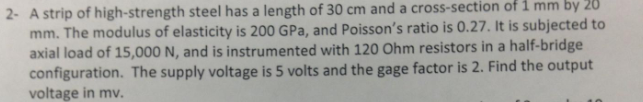A strip of high-strength steel has a length of 30 cm and a cross-section mm. The modulus of elasticity is 200 GPa, and Poisson's ratio is 0.27. It is subjected to axial load of 15,000 N, and is instrumented with 120 Ohm resistors in a half-bridge configuration. The supply voltage is 5 volts and the gage factor is 2. Find the output voltage in mv. of 1 by 20 mm 2- A strip of high-strength steel has a length of...

• ### G is 11.2*10^6 psi A shaft of length 2 m has the hollow cross section shown...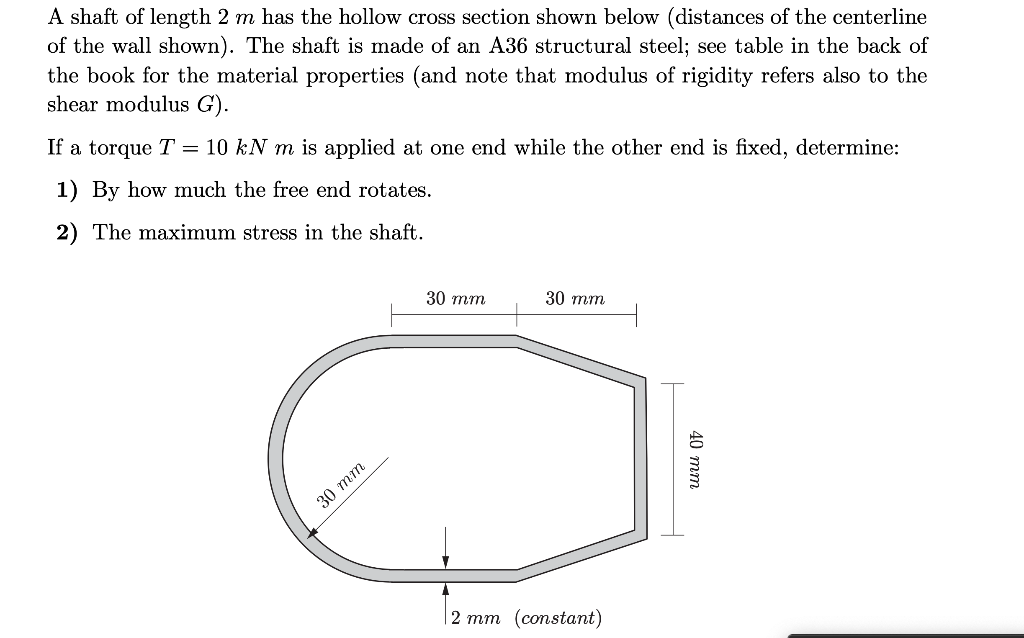G is 11.2*10^6 psi A shaft of length 2 m has the hollow cross section shown below (distances of the centerline of the wall shown). The shaft is made of an A36 structural steel; see table in the back of the book for the material properties (and note that modulus of rigidity refers also to the shear modulus G). If a torque T = 10 kN m is applied at one end while the other end is fixed, determine: 1)...

• ### The gears attached to the fixed-end steel shaft are subjected to the torques shown in the figure. If the shear modulus of elasticity is 150 GPa a determine the following: nd the shaft has a d...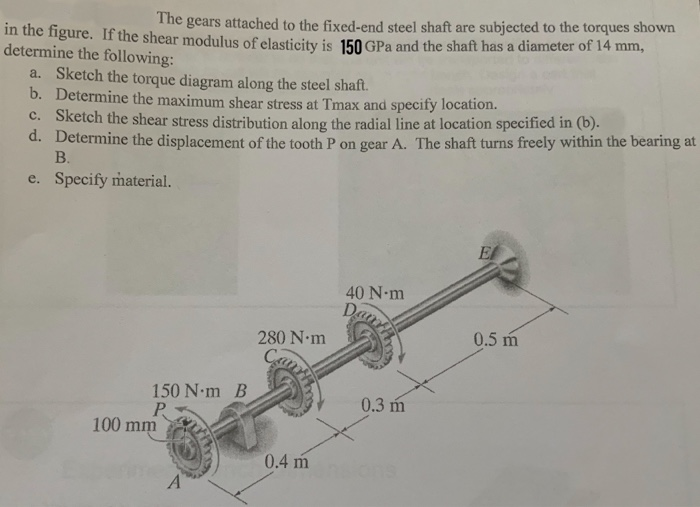The gears attached to the fixed-end steel shaft are subjected to the torques shown in the figure. If the shear modulus of elasticity is 150 GPa a determine the following: nd the shaft has a diameter of 14 mm, a. Sketch the torque diagram along the steel shaft. b. Determine the maximum shear stress at Tmax and specify location. c. Sketch the shear stress distribution along the radial line at location specified in (b). d. Determine the displacement of the...